# vs.eyeandcontacts.com

## Chapter 6 Lines and Angles Exercise 6.2

Question 1: In Fig. 6.28, find the values of x and y and then show that AB || CD.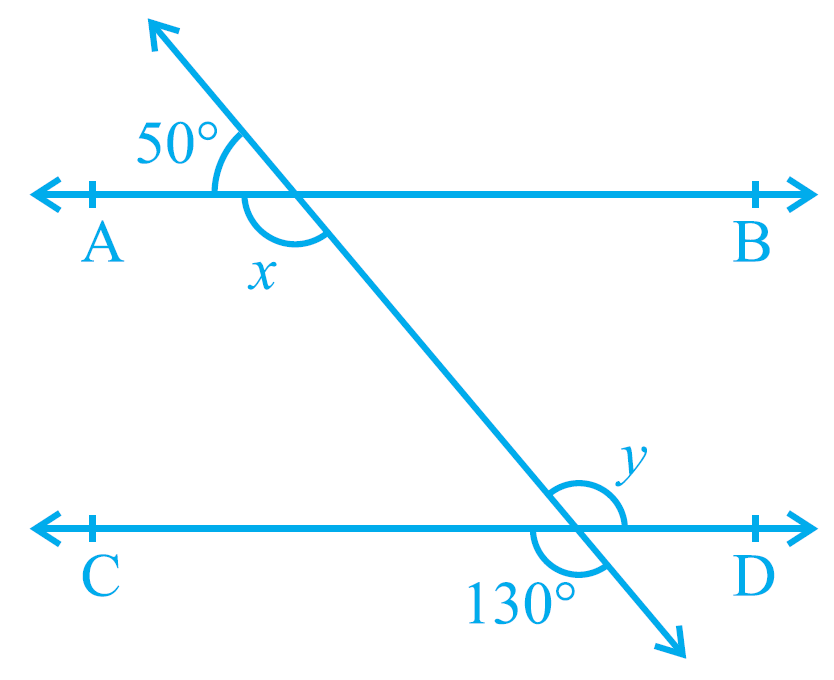∠y = 130° (Vertically Opposite Angles are equal)
x and 50° together form a linear pair.
So,
x + 50° = 180°
x = 180 - 50
x = 130°
x and y are alternate interior angles.
x = y
130° = 130°
As alternate interior angles are equal, the lines are parallel. Therefore, AB || CD.

Question 2: In Fig. 6.29, if AB || CD, CD || EF and y:z = 3:7, find x.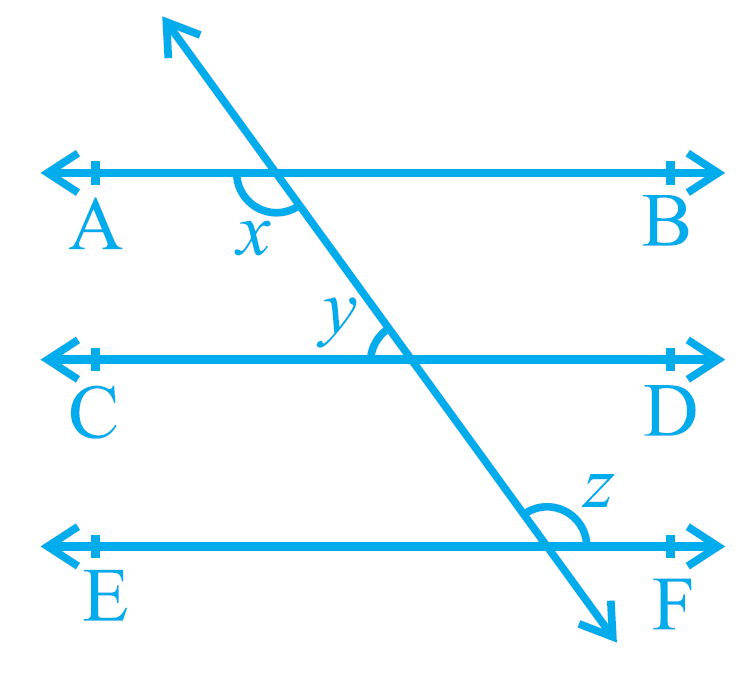Given
AB || CD
CD || EF
So, AB || EF
y:z = 3:7
Required to find
x
Steps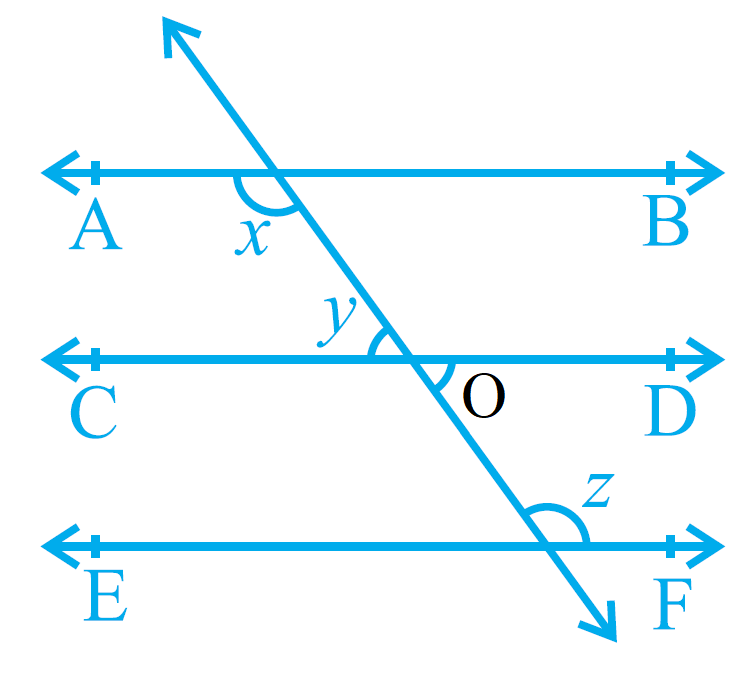x + y = 180° (Co-interior angles are supplementary)
x + z = 180° (Alternate interior angles are supplementary)
y = o (Vertically Opposite Angles are equal)
y + z = 180° (Co-interior angles are supplementary)
3a + 7a = 180°
10a = 180°
a = 180/10
a = 18°
y = 3a = 3 × 18 = 54°
z = 7a = 7 × 18 = 126°
x + y = 180°
x + 126° = 180°
x = 180 - 126
x = 54°
Therefore, x is 54°.

Question 3: In Fig. 6.30, if AB || CD, EF ⊥ CD and ∠GED = 126°, find ∠AGE, ∠GEF and ∠FGE.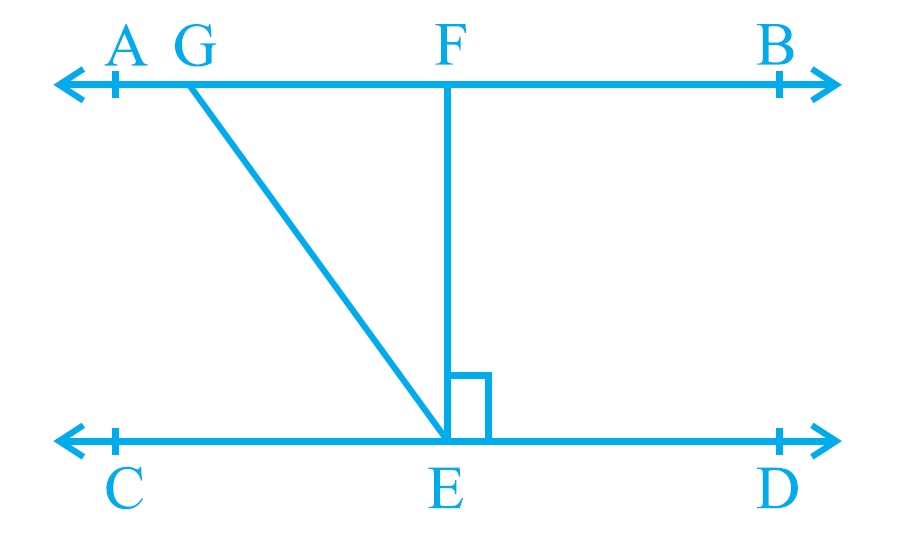Given
AB || CD
EF ⊥ CD
∠GED = 126°
Required to find
∠AGE
∠GEF
∠FGE
Solution
∠GEF = ∠GED - ∠FED
= 126° - 90°
= 36°
∠AGE = ∠GED (Alternate Interior Angles are equal)
∠AGE = 126°
∠AGE + ∠FGE = 180° (Linear pair are supplementary)
126° + ∠FGE = 180°
∠FGE = 180° - 126°
∠FGE = 54°
Therefore, ∠AGE is 126°, ∠GEF is 36° and ∠FGE is 54°.

Question 4: In Fig. 6.31, if PQ || ST, ∠PQR = 110° and ∠RST = 130°, find ∠QRS. [Hint: Draw a line parallel to ST through point R.]Given
PQ || ST
∠PQR = 110°
∠RST = 130°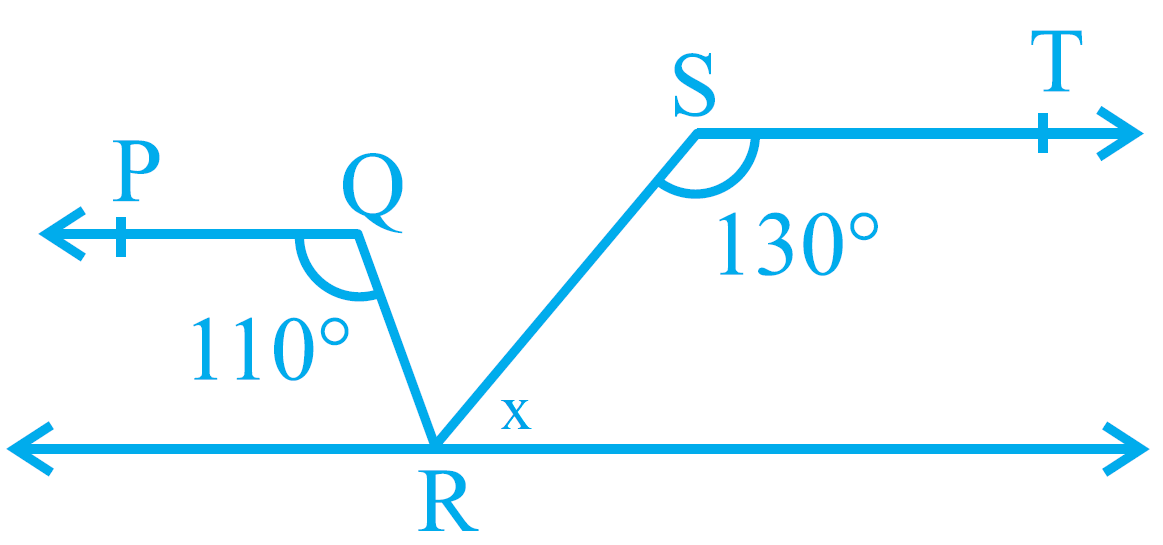Required to find
∠QRS
Solution
Line l is parallel to ST.
ST || PQ
QR is a transversal.
∠Q = ∠R (alternate interior angles are equal)
∠R = 110°
∠S + x = 180° (linear pair is supplementary)
130° + x = 180°
x = 180° - 130°
x = 50°
∠QRS = ∠R - x
= 110° - 50°
= 60°
Therefore, ∠QRS is 60°.

Question 5: In Fig. 6.32, if AB || CD, ∠APQ = 50° and ∠PRD = 127°, find x and y.Given
AB || CD
∠APQ = 50°
∠PRD = 127°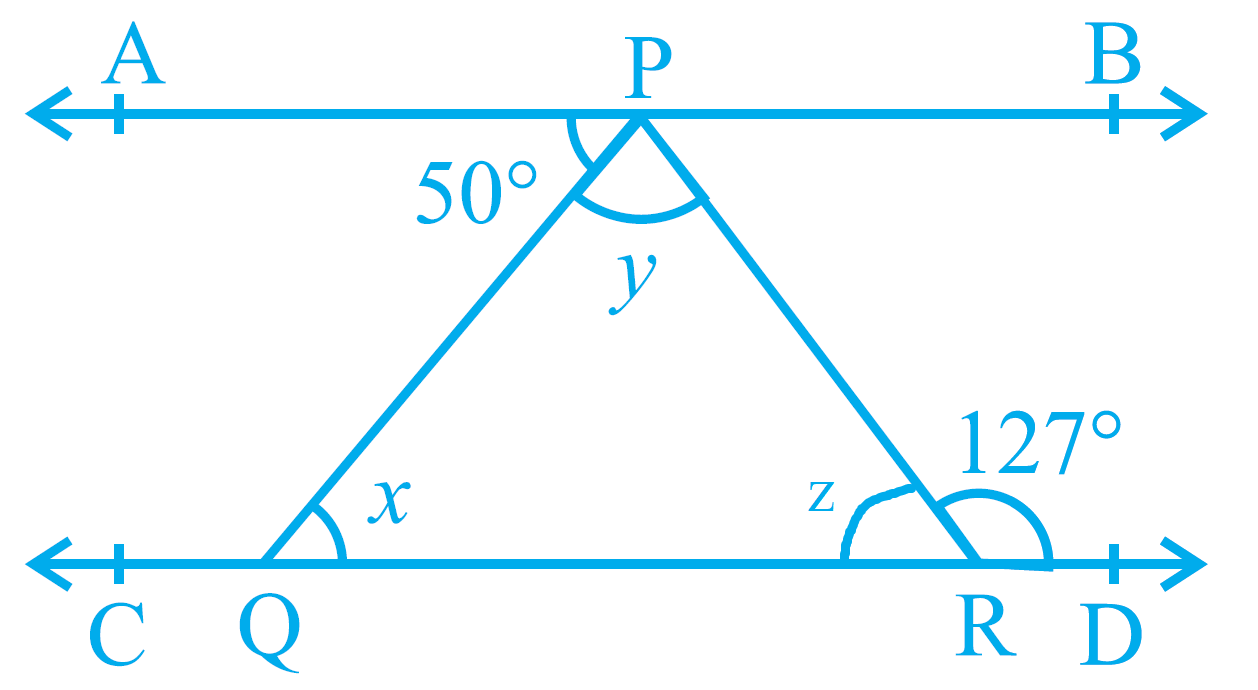Required to find
x and y
Solution
∠APQ = x (Alternate interior angles are equal)
x = 50°
∠PRD + z = 180 (linear pair are supplementary)
127° + z = 180°
z = 180° - 127°
z = 53°
x + y + z = 180° (Angle sum property of a triangle)
50° + y + 53° = 180°
103 + y = 180°
y = 180° - 103°
y = 77°
Therefore, x is 50° and y is 77°.

Question 6: In Fig. 6.33, PQ and RS are two mirrors placed parallel to each other. An incident ray AB strikes the mirror PQ at B, the reflected ray moves along the path BC and strikes the mirror RS at C and again reflects back along CD. Prove that AB || CD.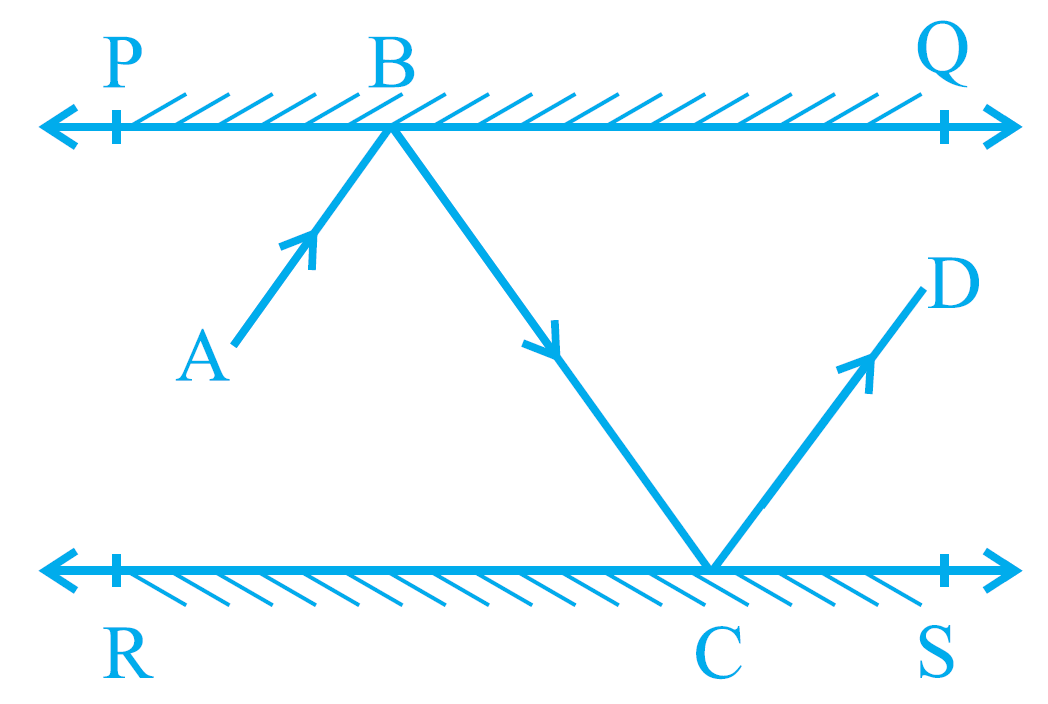Required to prove
AB || CD
Construction
Draw BM ⊥ PQ, CN ⊥ RS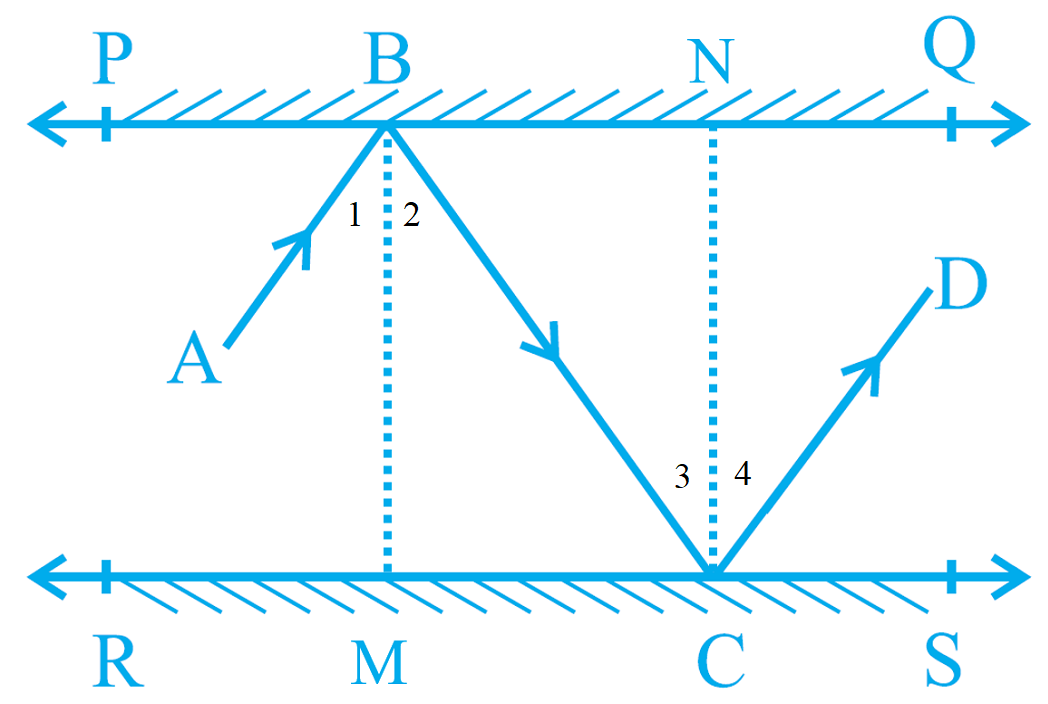Proof
Angle of incidence = Angle of reflection
∠1 = ∠2
∠3 = ∠4
Two line ⊥ to two || lines are parallel to each other.
Therefore, BM || CN
BC is the transversal.
Therefore, ∠2 = ∠3 (Interior alternate angles are equal).
So,
∠1 + ∠2 = ∠3 + ∠4 (Interior angles are equal)
Therefore, the lines are parallel i.e. AB || CD.